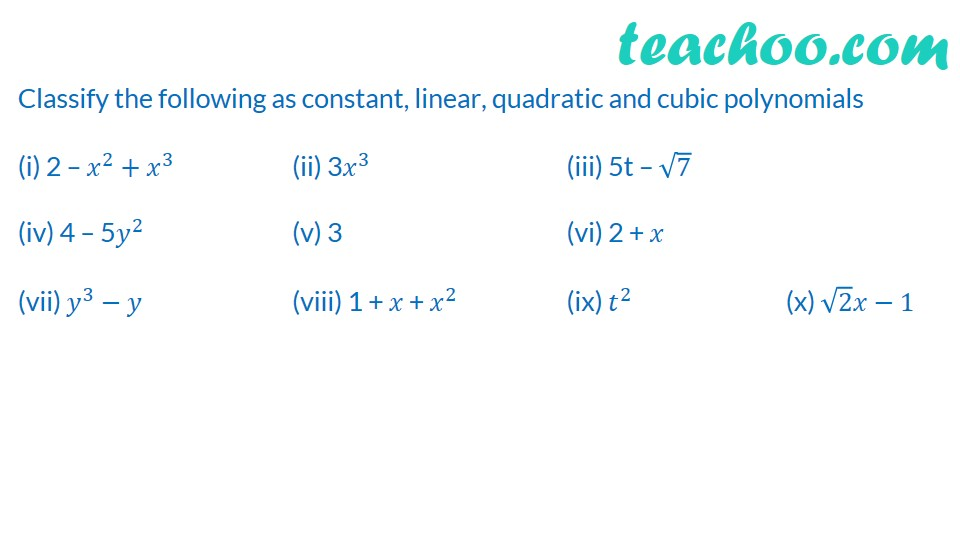Learn all Concepts of Polynomials Class 9 (with VIDEOS). Check - Polynomials Class 91. Chapter 2 Class 9 Polynomials
2. Concept wise
3. Degree & Coefficient of a polynomial

Transcript

Classify the following as constant, linear, quadratic and cubic polynomials (i) 2 – 𝑥^2+𝑥^3 (ii) 3𝑥^3 (iii) 5t – √7 (iv) 4 – 5𝑦^2 (v) 3 (vi) 2 + 𝑥 (vii) 𝑦^3−𝑦 (viii) 1 + 𝑥 + 𝑥^2 (ix) 𝑡^2 (x) √2 𝑥−1

Degree & Coefficient of a polynomial